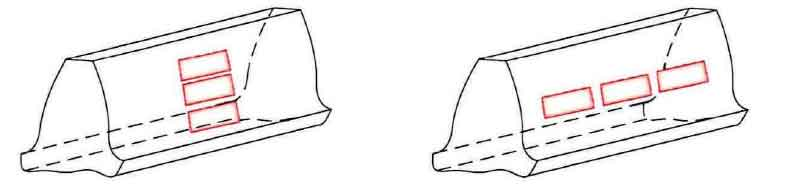# Multi pitting corrosion model of gear transmission system along tooth width direction

For as shown in figure 1 (b) along tooth width direction more corrosion characteristics of the model, select the multipoint corrosion x value range of tooth width direction x ∈ [Xs_st Xs_ed] is the same, for the convenience of analysis and discussion of definition of simulation conditions, still in three point corrosion pit, for example, four simulation conditions, simulation of different depth and width of the gear on the change of the time-varying mesh stiffness.(a) Along the tooth profile direction (b) Along the direction of tooth width

Simulation under the condition of time-varying mesh stiffness simulation results as shown in figure 2, figure 2 (a) under the condition of 1 in the time-varying mesh stiffness compared to no pitting characteristic stiffness value in the set point corrosion area presented to reduce the effect, still take a fixed-point erosion area under the average stiffness of stiffness of numerical comparison, its stiffness reduces the percentage of 5.85%. Similarly, the variation trend of stiffness curves under conditions 2 (Fig. 2 (b)), 3 (Fig. 2 (c)) and 4 (Fig. 2 (d)) is consistent with that in Fig. 2 (a), and the reduction percentage of stiffness in the preset pitting corrosion area is 3.25%, 5.83% and 3.23%, respectively. From the point of view of the percentage of stiffness reduction, the variable range of pitting pit width is larger and the effect of width on stiffness reduction is greater than that of depth on stiffness.